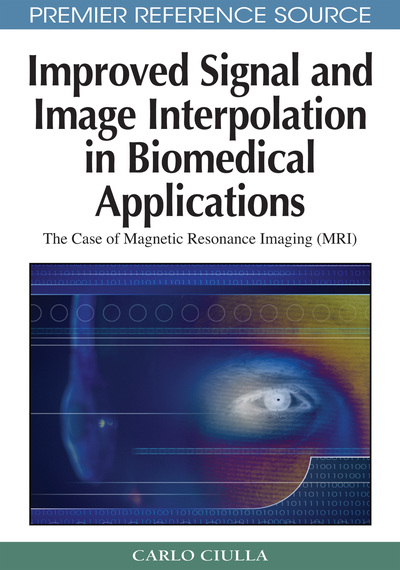# The Main Innovation Determined By the Sub-Pixel Efficacy Region

Carlo Ciulla (Lane College, USA)
DOI: 10.4018/978-1-60566-202-2.ch017
Available
\$37.50
No Current Special Offers

## Abstract

This chapter introduces the reader to Section V of the book. The chapter opens up with a discussion on the undeniable evidence reported in literature that the magnitude of the interpolation error is strictly related to the magnitude of the sampling resolution. While reference to the literature on the Lagrange interpolation function is reported elsewhere (Ciulla & Deek, 2006), the chapter devotes attention to the literature and the applications related to the Sinc function. The core of the chapter reports a section that condenses the message to the reader of this book about the main innovation determined through the Sub-pixel Efficacy Region. It is delivered to the reader the realization that combining signal intensity with the curvature of the interpolation function, the approximation properties of the model function can be improved. This message is linked to the bridging concept between classic and SRE-based interpolation which is that of the curvature of the interpolation function.
Chapter Preview
Top

## Literature

For a discussion on Lagrange approximation, literature and more generally on interpolation approximation error bounds the reader is referred to Ciulla and Deek (2006). For what concerns the present chapter it is necessary to recall that the literature extensively admits and reports the evidence that the goodness of approximation of any interpolation function is inversely proportional to the sampling step (resolution). Conceptually this is straightforward since the degree of uncertainty of the estimate of the model interpolation increases proportionally to the length of the curve connecting two adjacent nodes. That is to say that the degree of uncertainty about the true local curvature between two adjacent nodes increases with the increase of the sampling step. Such increase is detrimental because of two fundamental reasons. Both reasons descend from the nature of the ill posed problem that the model interpolator attempt to solve. The first and main reason is that the true signal is unknown and from this concept it descends that the true local curvature is consequentially unknown. The second reason is consequential to the first one and it reveals itself by the nature of the task the interpolator has to perform which is that of approximation. To approximate within a large sampling step is thus far more difficult thus prone to a larger error because the length of the curve that the model interpolator has to reconstruct is extended. This concept applies in either image space or k-space (frequency domain).

Nevertheless, as demonstrated by early experimental research (Whittaker, 1915, 1935), the ideal approach to interpolation remains the Sinc interpolation function. The reason why Sinc interpolation proves to be superior stands in its theoretical background as it considers the signal to be defined from -∞ to +∞ through infinite convolution of the sample node intensities. The typical Sinc function is: ∞ ∞ x (t) = Σ -∞ x (nT) sinc(π(t-nT)/T) = Σ -∞ x (nT) sin[ (π(t-nT)/T) ] / (π(t-nT)/T) (1) where T is the sampling period. Two main limitations arise from equation (1) and they are: (i) the number of intensity values (nT) are finite, and (ii) values of the sampling point close or equal to t = nT furnish an undetermined expression of the type 0/0. Methods of approximating the Sinc interpolation function given by equation (1) through a finite summation of samples have been explored (Schanze, 1995). It was also demonstrated that the approximated form of the Sinc function can also be represented by the Lagrange function (Whittaker, 1915, 1935).

Schanze (1995) reported the following expressions of the finite sum approximation of the Sinc function: M –1 x (t) = sin[ πt / N ] * Σn = - L xn (-1n) cot [π (t - n)/ N], N even (2) M –1 x (t) = sin[ πt / N ] * Σn = - L xn (-1n) csc [π (t - n)/ N], N odd (3) Alternative formulations were reported (Candocia & Principe, 1998; Dooley & Nandi, 2000).

## Complete Chapter List

Search this Book:
Reset